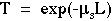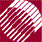ECE532 Biomedical Optics © 1998 Steven L. Jacques, Scott A. Prahl Oregon Graduate Institute

# Definition and units of scattering coefficient

Consider a scattering particle idealized as a sphere with a particular geometrical size. Consider that this sphere redirects incident photons into new directions and so prevents the forward on-axis transmission of photons, thereby casting a shadow. This process constitutes scattering. This description is of course an oversimplified and schematicized version of the real situation. However, it does provide a simple concept which captures the essence of the scattering coefficient, a parameter analogous to the absorption coefficient discussed previously.

The size of the scattering shadow is called the effective cross-sections [cm2]) and can be smaller or larger than the geometrical size of the scattering particle (A [cm2]), related by the proportionality constant called the scattering efficiency Qs [dimensionless]: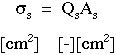The scattering coefficient µs [cm-1] describes a medium containing many scattering particles at a concentration described as a volume densitys [cm3]. The scattering coefficient is essentially the cross-sectional area per unit volume of medium.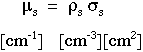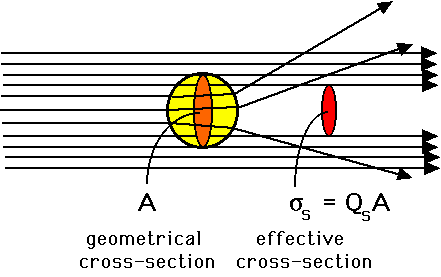Experimentally, the units [cm-1] for µs are inverse length, such that the product µsL is dimensionless, where L [cm] is a photon's pathlength of travel through the medium. The probability of transmission T of the photon without redirection by scattering after a pathlength L is: